Electricity and Magnetism

Series and parallel resistors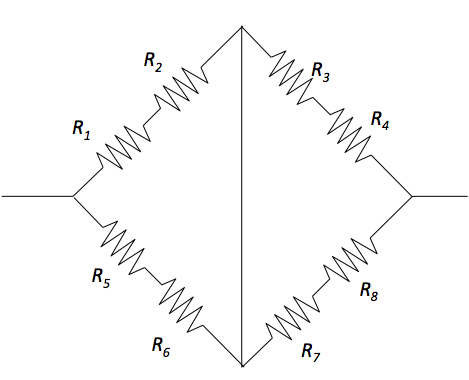Given $R_1 = 3.0\ \Omega,$ $R_2 = 6.0\ \Omega,$ $R_3 = 4.0\ \Omega,$ $R_4 = 8.0\ \Omega,$ $R_5 = 6.0\ \Omega,$ $R_6 = 3.0\ \Omega,$ $R_7 = 6.0\ \Omega$ and $R_8 = 12.0\ \Omega,$ what is the approximate overall equivalent resistance?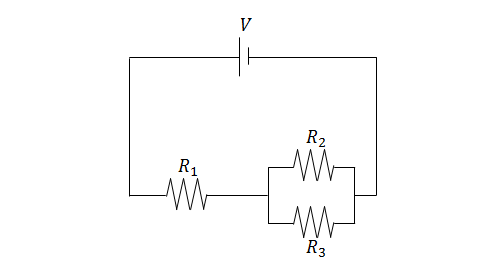In the circuit above, $V=9.6\text{ V},$ $R_1=2\ \Omega,$ $R_2=9\ \Omega,$ and $R_3=18\ \Omega.$ Find the current through $R_3.$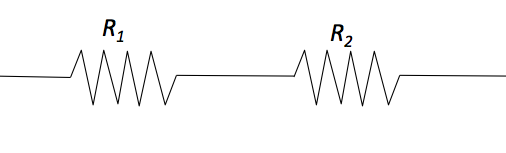Given $R_1 = 5\ \Omega$ and $R_2 = 10\ \Omega,$ what is the overall equivalent resistance?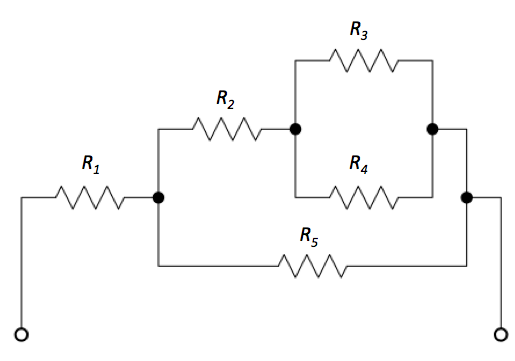Given $R_1 = 5.0\ \Omega,$ $R_2 = 4.0\ \Omega,$ $R_3 = 2.0\ \Omega,$ $R_4 = 6.0\ \Omega,$ and $R_5 = 8.0\ \Omega,$ what is the approximate overall equivalent resistance?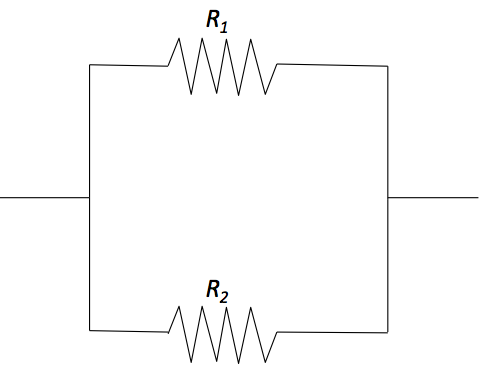Given $R_1 = 9\ \Omega$ and $R_2 = 18\ \Omega,$ what is the overall equivalent resistance?

×